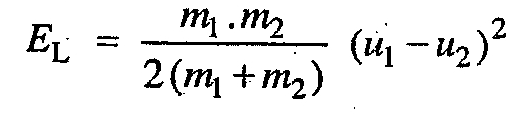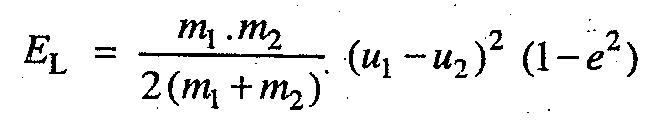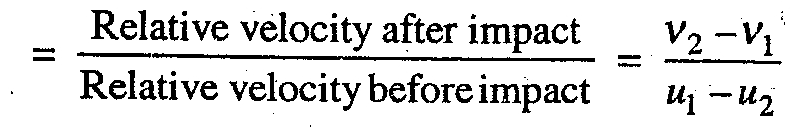### Elastic and Inelastic Collisions

Understanding conservation of energy using collision of elastic and inelastic bodies

Consider the impact between two bodies which move with different velocities along the same straight line. It is assumed that the point of impact lies on the line joining the centers of gravity of the two bodies. The behavior of these colliding bodies during the complete period of impact will depend upon the properties of the materials of which they are made. The material of the two bodies may be perfectly elastic or perfectly inelastic.

The bodies which rebound after impact are called elastic bodies and the bodies which does not rebound at all after its impact are called inelastic bodies. The impact between two lead spheres or two clay spheres is approximately an inelastic impact.

The loss of kinetic energy (EL) during impact of inelastic bodies is given bywhere

m1 = Mass of the first body,
m2 = Mass of the second body,
u and u2 = Velocities of the first and second bodies respectively.

The loss of kinetic energy (EL) during impact of elastic bodies is given bywhere
e = Coefficient of restitution.Notes:

1. The relative velocity of two bodies after impact is always less than the relative velocity before impact.

2. The value of e=0, for perfectly inelastic bodies and e=1, for perfectly elastic bodies. In case the bodies are neither perfectly inelastic nor perfectly elastic, then the value of e lies between zero and one.